If you have a related question, please click the "Ask a related question" button in the top right corner. The newly created question will be automatically linked to this question.

• Resolved

# XTR106: input voltage of XTR106 and design based on datasheet example

Part Number: XTR106

Hi

I have to design a 4-20 mA transmitter circuit for a load cell with about 520ohm impedance.

I used XTR106 and circuit that mentioned in figure 5 of XTR106 datasheet.

so I used the circuit like figure 5 of datasheet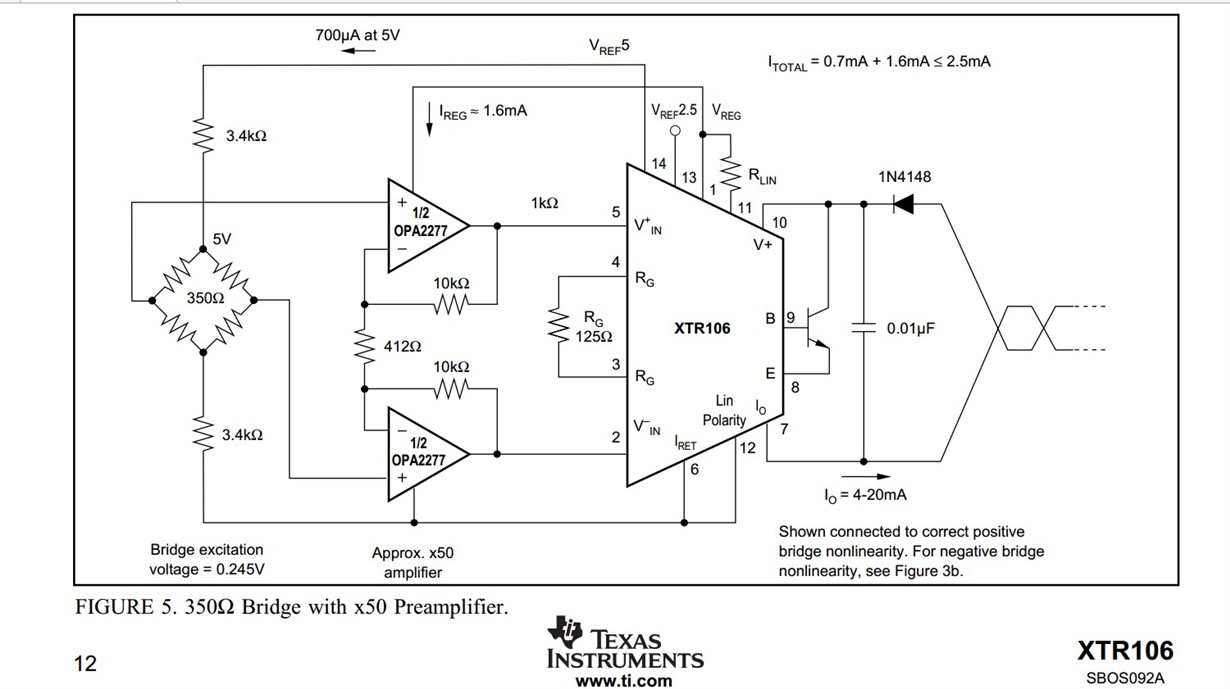I think the input range of XTR106 have to be between 1.1 and 3.5V.

but in this example I think the characteristic value is about 4.0817 mV/V so the input full scale voltage is 0.245*4.0817*50=50 mV

so the full scale current is Io=(50*40/125)+4=20

in this example the input voltage of XTR106 is betwwen 0 and 50 mV is it OK?

for my application I have a load pin sensor with

Zero signal: -0.0009 mV / V
Characteristic value: 1.0147 mV / V

so I used this circuit for it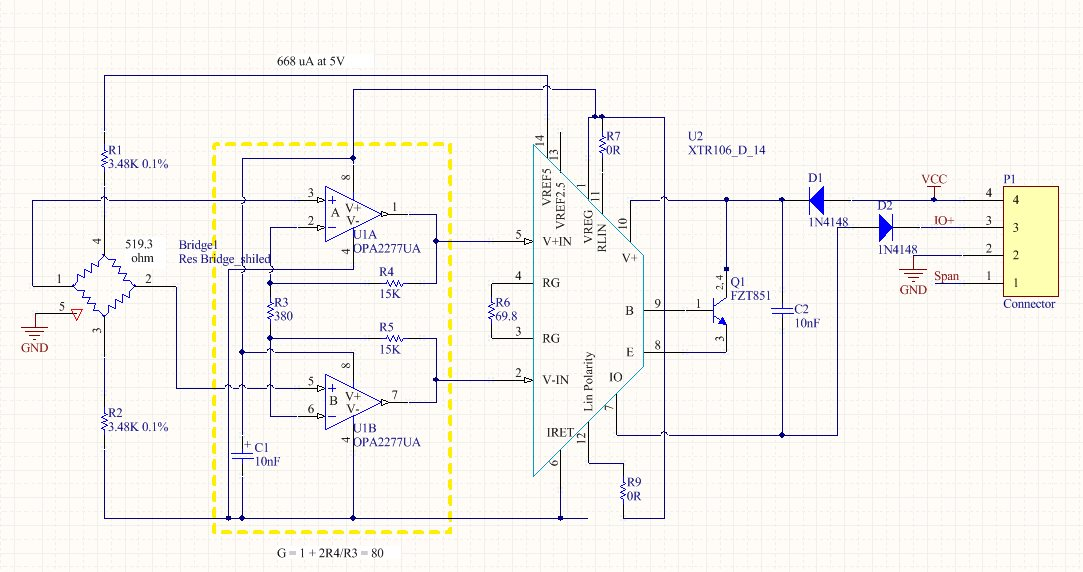but for this circuit I have less than 4 mA at the first!

is my design ok? How I can solve my problem?

• Hi Amir,

the bridge excitation voltage of 0.245V has nothing to do with the output signal of XTR106. It's only the voltage that drops across the whole bridge. This voltage drop is of interest, if bridge heating is an issue.

Kai
• In reply to kai klaas69:

Hi Kai

we have relation between Vfs and Vexcitation. so 0.245V is important for the output current! is my computing true for the figure 5 or I understood somthing wrong?

Vcc=24V

if I remove the bridge or R6 I have constant 4mA current so XTR106 work correctly. but when I connect the bridge and R6 the output current is about 2.6mA. I measured the input voltage it was negative. I think it's my problem.
is it everything right for my design?

Hi Amir,

why is the input voltage negative? How have you measured this?

What is the full scale output signal of bridge? 1.0147 mV/V? Can you show a datasheet of your bridge?

Kai
• In reply to kai klaas69:

Hi kai

I think because of sensor's zero signal

I measured the voltage between pin 5 and pin 2 of Xtr106

yes it's 1.0147 mV/V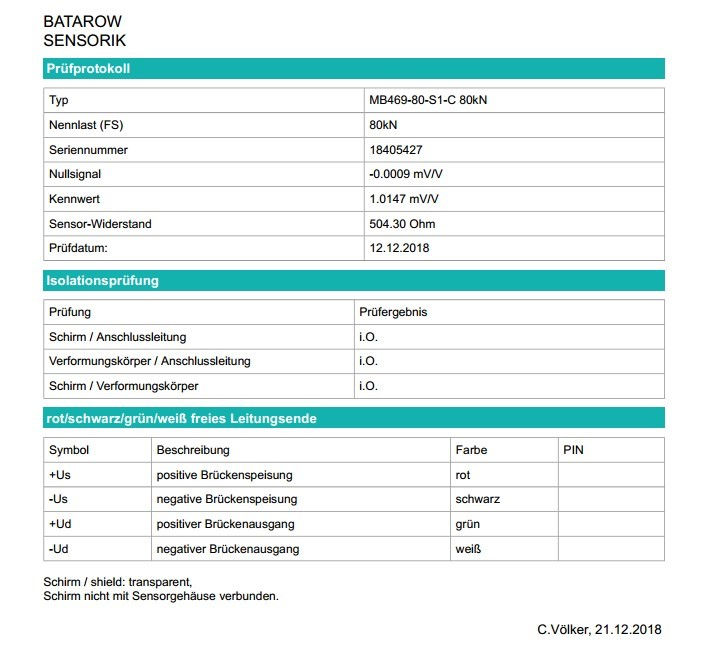I have about -7mv between pin 5 and pin 2 of XTR106 and the output current is about 2.9 mA for zero situation.

if I put a pressure in my load pin the voltage would be changed so it's ok but the output current is constant!

Hi Amir,

have you seen that the datasheet of XTR106 recommends the use of an offset adjust scheme?

e2e.ti.com/.../680598

Kai
• In reply to kai klaas69:

Hi Kai

I read it but I don't know what changes in my circuit can solve the problem.

Hi Amir,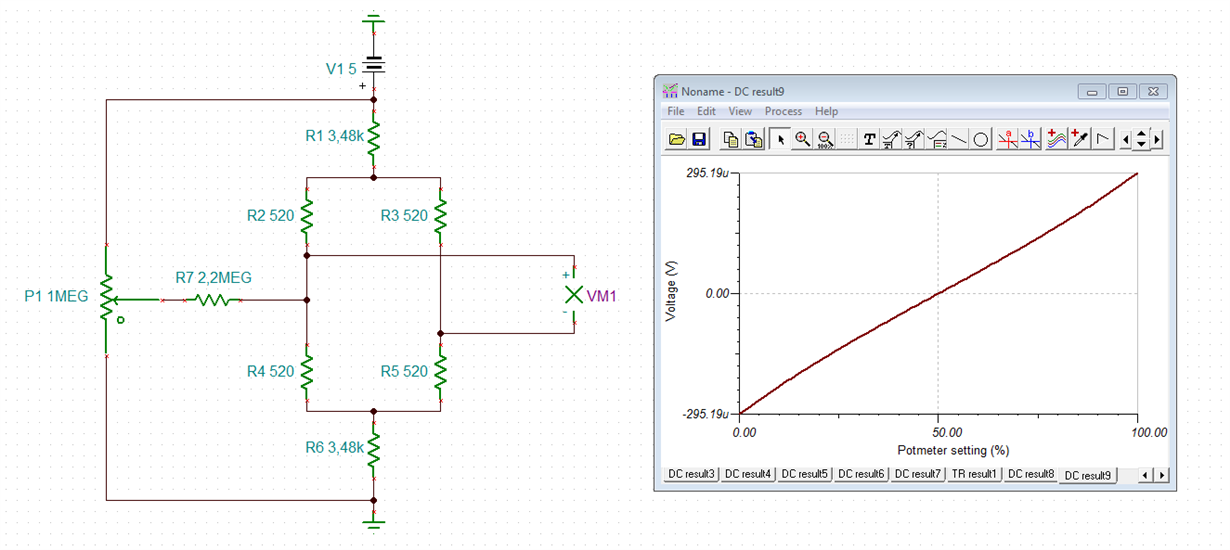Or use a scheme like that, to decrease the adjustment range: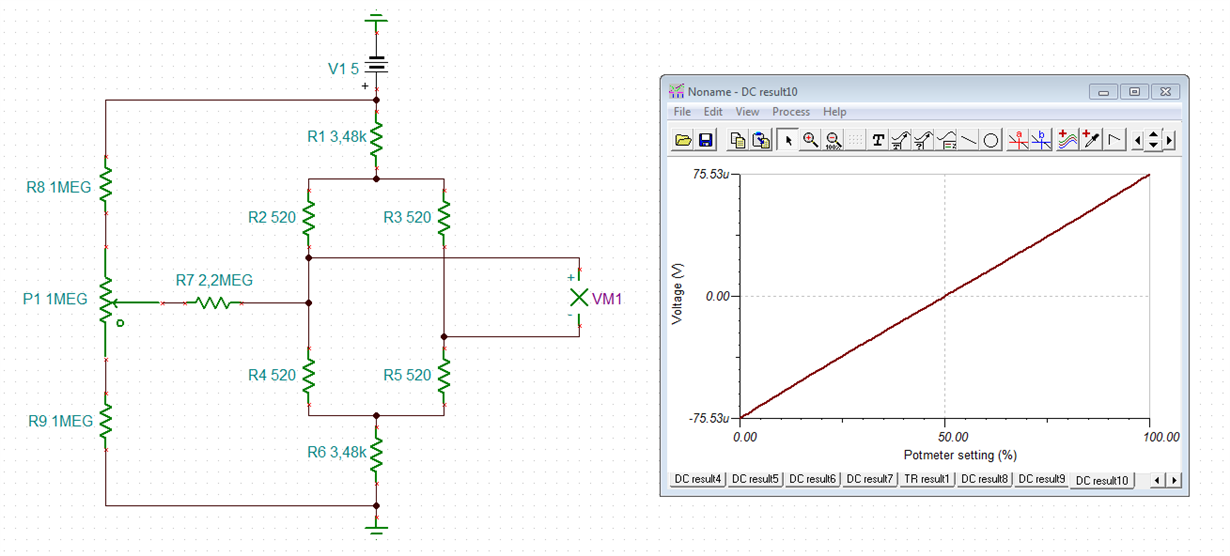amir.TSC

Have these additional components well shielded and use low pass filtering at the inputs of OPA2277 as shown in figure 6 of datasheet of XTR106.

Kai

Hi Amir,

does it work now?

Kai
• In reply to kai klaas69:

Hi Kai

I'm in travel now

I will tell you the results any time that I test it